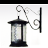# Tool for a moving average over a time series

4480
5
09-07-2015 05:50 AMNew Contributor III

Dear all,

I am looking for a tool to create a pixel-based moving average from a range of MODIS EVI tiles (from 2001 to 2013).

Is there a tool in ArcGIS to create the respective raster data sets by aggregating for example 3 time steps?

Stefanie

Tags (4)
5 RepliesbyMVP Esteemed Contributor

If you are talking moving average as being the time step for each spatial location, then have you seen

http://desktop.arcgis.com/en/desktop/latest/tools/spatial-analyst-toolbox/how-cell-statistics-works....

and the mean example.  It will give you the average at each location given rasters of the same extent, cell size just representing different times.

If you want a 'moving' average at one time step, then you want to look at

http://desktop.arcgis.com/en/desktop/latest/tools/spatial-analyst-toolbox/focal-statistics.htm

you could use a 3x3 window and calculate the average within the 9 cells.  Depending how the average is calculated for a window, this may yield the same results.

An example using simple mean calculations (and I had Python open...sorry)

```>>> import numpy as np
>>> a = np.arange(9.).reshape((3,3))
>>> b = np.arange(5.,14.).reshape((3,3))
>>> c = np.arange(10.,19.).reshape((3,3))
>>> a_m = np.mean(a)
>>> b_m = np.mean(b)
>>> c_m = np.mean(c)
>>> cent_m = np.mean([a_m,b_m,c_m])
>>> cent_m
9.0
>>> all_data = np.array([a,b,c])
>>> all_data = all_data.flatten()
>>> all_data_mean = np.mean(all_data)
>>> all_data_mean
9.0
>>> a  # time step 1
array([[ 0.,  1.,  2.],
[ 3.,  4.,  5.],
[ 6.,  7.,  8.]])
>>> b  # time step 2
array([[  5.,  6.,  7.],
[  8.,  9.,  10.],
[ 11.,  12.,  13.]])
>>> c  # time step 3
array([[ 10.,  11.,  12.],
[ 13.,  14.,  15.],
[ 16.,  17.,  18.]])
>>> a_m, b_m, c_m  # means for arrays a, b, c
(4.0, 9.0, 14.0)
>>> all_data_mean  # mean of flattened array of all data,
9.0
>>>```

Just some food for thought

EDIT

Oh yeah... of course you can do this within python.  You can get your ArcMap data layers into numpy array format using

http://desktop.arcgis.com/en/desktop/latest/analyze/arcpy-functions/rastertonumpyarray-function.htm

and you could simply create a multi-dimensional array from the inputs, or work with each layer one at a time.  It is trivial to setup and there are workarounds for humungous data files etc (ie netCDF support)New Contributor III

Hi Dan,

I am not sure if what you edited meets what I thought as I am really not familiar with Python programming and can't read the code.

Concerning the other two options - I am not looking for the averages over all time steps, nor for a moving window filter for one. What I am seeking is something that can easily calculate the mean for each cell for layer 1, 2 and 3, then one for 2, 3 and 4, the next one for 3, 4, and 5 etc. I know I could simply do it with the raster calculator, but since I have a large number of rasters, this will take ages to do manually.byMVP Esteemed Contributor

What you want is to do is code the local statistics in a moving loop... sorry to stick to code but ultimately you want something like this

```>>> import numpy as np
>>>
>>> rasters = np.array([1,2,3,4,5,6,7,8,9])
>>> moving_mean = [np.mean(rasters[i-3:i]) for i in range(3,len(rasters)+1)]
>>> moving_mean
[2.0, 3.0, 4.0, 5.0, 6.0, 7.0, 8.0]
>>>```

but using something like this totally unchecked code (money refunded if it doesn't work)

```arcpy.CheckOutExtension("Spatial")
env.workspace = "C:/somepath/to_your/data"
rasters = arcpy.ListRasters("*", "GRID")
ras_name = "amazing"
for i in range(3,len(rasters)+1):
outras = CellStatistics([rasters[i-3],rasters[i-2],rasters[i-1]], "Mean", "NODATA")
ras_name = env.workspace + "/" +ras_name + str(I)
ras_name.save(ras_name)```

Now maybe someone with some data, or is so inclined could check this out.New Contributor III

Thanks for the code, I'll try it on my data and will give you feedback!

I guess there's no way around Python when seriously working with ArcGIS..byMVP Esteemed Contributor

No...It is important to learn as many languages as possible...there isn't a code Bablefish however.